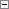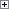A compound statement is one type of control structure in C/AL.

Using Compound Statements

In some cases, the C/AL syntax only lets you use a single statement. However, if you have to execute more than one simple statement, the statements can be written as a compound statement by enclosing the statements between the BEGIN and END keywords.Copy Code
BEGIN
<Statement 1>;
<Statement 2>;
..
<Statement n>;
END

The individual statements are separated by a semicolon. In C/AL, a semicolon is used to separate statements and not, as in other programming languages, as a terminator symbol for a statement. Nevertheless, an extra semicolon before an END does not cause an error because it is interpreted by the compiler as an empty statement.

Blocks

The BEGIN-END structure is also called a block. Blocks can be very useful to refer to the other control structures in C/AL.

When BEGIN follows THEN, ELSE, or DO, it should be on the same line and preceded by one space character.

ExampleCopy Code
IF (x = y) AND (a = b) THEN BEGIN
x := a;
y := b;
END;

ExampleCopy Code
IF (xxx = yyyyyyyyyy) AND
(aaaaaaaaaa = bbb)
THEN BEGIN
x := a;
x := y;
a := y;
END ELSE BEGIN
y := x;
y := a;
END;